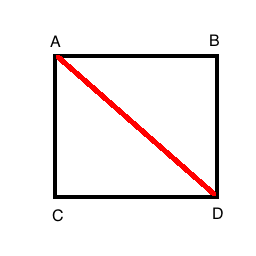January 24th, 2013

Filed under: OpenGL | Be the first to comment!

When writing a 2D game in OpenGL, you generally draw texture-mapped quads. The most straightforward way to draw a texture-mapped quad with vertex arrays is to supply GL_QUADS as the first argument to glDrawArrays(). But GL_QUADS is not available on OpenGL ES so if you want to port your game to mobile devices you can’t use GL_QUADS. Also, GL_QUADS was deprecated in OpenGL 3 so using GL_QUADS is not wise if you’re writing a new OpenGL application. What is the replacement for GL_QUADS?

The simplest replacement is to use GL_TRIANGLES. If you look at the following figure:You can see that drawing a line from A to D (or from B to C) splits the rectangle into two triangles. Add the vertices for the two triangles and use GL_TRIANGLES to draw the quad.

The downside of using GL_TRIANGLES is that it requires six vertices to draw a quad instead of four. How you order the vertices is up to you, but the following order works for me:

Vertex 1: C (lower left)

Vertex 2: A (upper left)

Vertex 3: D (lower right)

Vertex 4: D (lower right)

Vertex 5: B (upper right)

Vertex 6: A (upper left)